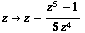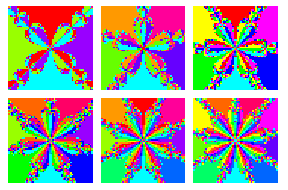# Newton’s Method

The following figure shows the basins of attraction for z → z5−1 using Newton’s method in the complex plane:Here’s the code (we find the fixed point ofwhich should land on a solution to z5 = 1, for a 125-by-125 array of complex starting values between −1.5 − 1.5 i and 1.5 + 1.5 i; we color each point on the array according to the solution that Newton’s method ends with, or rather the phase of the ending point, which amounts to the same thing):

```theFunction[z_] := z^5;

iterateThis[z_] = z - (theFunction[z] - 1)/theFunction'[z];

(* at the moment, the graph is backwards, but I can live with that *)

ContourPlot[
Arg[
FixedPoint[ iterateThis, x + I y, 30 ]],
{x, -1.5, 1.5}, {y, -1.5, 1.5},
ContourLines -> False, PlotPoints -> 125,
ColorFunction -> Hue, Axes -> False, Frame -> False ];```

Using the enhanced `Compile` function in Mathematica version 3.0, we can make this roughly a zillion times faster:

```(* works just for z^n = 1, integer n > 2;
should be a snap to modify it to work with any poly. function,
but I have to get back to work now *)

newt =
Compile[{{n, _Integer}, {z, _Complex}},
Arg[ FixedPoint[# - (#^n - 1)/(n #^(n - 1))&, z, 35] ]];

Show[
GraphicsArray[
Partition[
Table[
ListDensityPlot[Table[newt[n, b + a I],
{a, -1.1, 1.1, 0.03}, {b, 1.1, -1.1, -0.03}],
Mesh -> False, ColorFunction -> Hue, Frame -> False,
DisplayFunction -> Identity],
{n, 4, 12}],
3],
GraphicsSpacing -> 0.05]]```

More of the same:Designed and rendered too long ago using Mathematica 3.0 for the Apple Macintosh.

Fame, esoteric and imagined: The previous image was used on the cover of The Mathematica Journal, issue 7, volume 1.